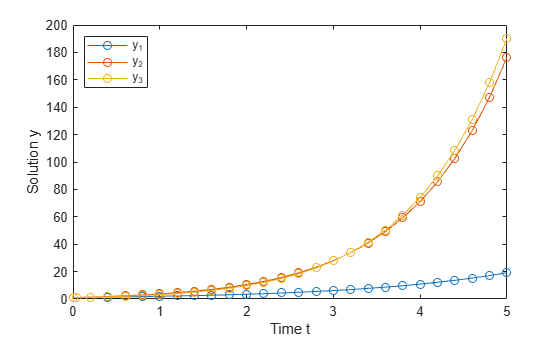Main Content

# DDE with Constant Delays

This example shows how to use `dde23` to solve a system of DDEs (delay differential equations) with constant delays.

The system of equations is

`$\begin{array}{l}{{\mathit{y}}_{1}}^{\prime }\left(\mathit{t}\right)={\mathit{y}}_{1}\left(\mathit{t}-1\right)\\ {{\mathit{y}}_{2}}^{\prime }\left(\mathit{t}\right)={\mathit{y}}_{1}\left(\mathit{t}-1\right)+{\mathit{y}}_{2}\left(\mathit{t}-0.2\right)\\ {{\mathit{y}}_{3}}^{\prime }\left(\mathit{t}\right)={\mathit{y}}_{2}\left(\mathit{t}\right).\end{array}$`

The history function for $\mathit{t}\le 0$ is constant, ${\mathit{y}}_{1}\left(\mathit{t}\right)={\mathit{y}}_{2}\left(\mathit{t}\right)={\mathit{y}}_{3}\left(\mathit{t}\right)=1$.

The time delays in the equations are only present in $\mathit{y}$ terms, and the delays themselves are constants, so the equations form a system of constant delay equations.

To solve this system of equations in MATLAB, you need to code the equations, delays, and history before calling the delay differential equation solver `dde23`, which is meant for systems with constant delays. You either can include the required functions as local functions at the end of a file (as done here), or save them as separate, named files in a directory on the MATLAB path.

### Code Delays

First, create a vector to define the delays in the system of equations. This system has two different delays:

• A delay of 1 in the first component ${\mathit{y}}_{1}\left(\mathit{t}-1\right)$.

• A delay of 0.2 in the second component ${\mathit{y}}_{2}\left(\mathit{t}-0.2\right)$.

`dde23` accepts a vector argument for the delays, where each element is the constant delay for one component.

`lags = [1 0.2];`

### Code Equation

Now, create a function to code the equations. This function should have the signature `dydt = ddefun(t,y,Z)`, where:

• `t` is time (independent variable).

• `y` is the solution (dependent variable).

• `Z(:,j)` approximates the delay $\mathit{y}\left(\mathit{t}-{\tau }_{\mathit{j}}\right)$, where the constant delay ${\tau }_{\mathit{j}}$ is given by `lags(j)`.

These inputs are automatically passed to the function by the solver, but the variable names determine how you code the equations. In this case:

• `Z(:,1)`$\text{\hspace{0.17em}}\to \text{\hspace{0.17em}}{\text{\hspace{0.17em}}\mathit{y}}_{1}\left(\mathit{t}-1\right)$

• `Z(:,2)`$\text{\hspace{0.17em}}\to {\text{\hspace{0.17em}}\text{\hspace{0.17em}}\mathit{y}}_{2}\left(\mathit{t}-0.2\right)$

```function dydt = ddefun(t,y,Z) ylag1 = Z(:,1); ylag2 = Z(:,2); dydt = [ylag1(1); ylag1(1)+ylag2(2); y(2)]; end ```

Note: All functions are included as local functions at the end of the example.

### Code Solution History

Next, create a function to define the solution history. The solution history is the solution for times $\mathit{t}\le {\mathit{t}}_{0}$.

```function s = history(t) s = ones(3,1); end ```

### Solve Equation

Finally, define the interval of integration $\left[{\mathit{t}}_{0}\text{\hspace{0.17em}}{\text{\hspace{0.17em}}\mathit{t}}_{\mathit{f}}\right]$ and solve the DDE using the `dde23` solver.

```tspan = [0 5]; sol = dde23(@ddefun, lags, @history, tspan);```

### Plot Solution

The solution structure `sol` has the fields `sol.x` and `sol.y` that contain the internal time steps taken by the solver and corresponding solutions at those times. (If you need the solution at specific points, you can use `deval` to evaluate the solution at the specific points.)

Plot the three solution components against time.

```plot(sol.x,sol.y,'-o') xlabel('Time t'); ylabel('Solution y'); legend('y_1','y_2','y_3','Location','NorthWest');```### Local Functions

Listed here are the local helper functions that the DDE solver `dde23` calls to calculate the solution. Alternatively, you can save these functions as their own files in a directory on the MATLAB path.

```function dydt = ddefun(t,y,Z) % equation being solved ylag1 = Z(:,1); ylag2 = Z(:,2); dydt = [ylag1(1); ylag1(1)+ylag2(2); y(2)]; end %------------------------------------------- function s = history(t) % history function for t <= 0 s = ones(3,1); end %-------------------------------------------```

Download ebook